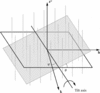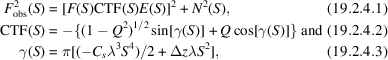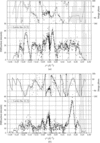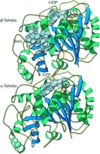International
Tables for
Crystallography
Volume F
Crystallography of biological macromolecules
Edited by M. G. Rossmann and E. Arnold

International Tables for Crystallography (2006). Vol. F, ch. 19.2, pp. 425-427   | 1 | 2 |

Section 19.2.4. Data processing

W. Chiua*

aVerna and Marrs McLean Department of Biochemistry and Molecular Biology, Baylor College of Medicine, Houston, Texas 77030, USA
Correspondence e-mail: wah@bcm.tmc.edu

19.2.4. Data processing

| top | pdf |

19.2.4.1. Data sampling

| top | pdf |

The principle of three-dimensional reconstruction is based on the central section theorem, which states that the experimental or computed projected diffraction pattern of a three-dimensional object is a plane that intersects the centre of the three-dimensional Fourier space in the direction normal to the direction of the projection (DeRosier & Klug, 1968). Because of the crystallographic symmetry inherent in a protein crystal, only a portion of the entire three-dimensional Fourier space, equivalent to an asymmetric unit of the crystal unit cell, is needed for the reconstruction. The structure factors of a three-dimensional crystal are localized in the three-dimensional reciprocal lattice, whereas the structure factors of a two-dimensional crystal are distributed continuously along the lattice lines, each of which passes through the reciprocal lattice in the zero projection plane (Fig. 19.2.4.1)(Henderson & Unwin, 1975). The assignment offor each observation (h, k,) along the lattice line is determined from the tilt angle and direction of the tilt axis for each image (Shaw & Hills, 1981). In general, the three-dimensional data set is initially built up from low-angle data and is gradually extended to the high-angle data. The angular parameters for each observed reflection are iteratively refined among one another within the whole data set. The required accuracy of these angular determinations depends on the thickness of the crystal and also on the desired resolution (Prasad et al., 1990). For instance, the angular accuracy has to be < 0.1° for 3.5 Å data in a 100 Å-thick crystal. The sampling of the data along the lattice line is generally aboutthe thickness of the crystal (Henderson et al., 1990). The data points are not evenly sampled along the lattice lines; they must be fitted into continuous and smoothly varying functions within the constraint of the crystal thickness. These functions are interpolated onto a periodic lattice, so that its inverse Fourier transform can be computed to reconstruct the three-dimensional mass-density function of the object.Figure 19.2.4.1 | top | pdf |Schematic diagram of data distribution in Fourier space for a two-dimensional crystal. Both the amplitudes and phases of the structure factors are distributed along the lattice lines passing through the (h, k) projection plane. The sectional plane shown denotes data from a crystal tilted at a certain angle (ϕ), and its tilt axis has an inclined angle (θ) with respect to one of its crystallographic axes. (Courtesy of Dr Jaap Brink at Baylor College of Medicine.)

19.2.4.2. Amplitudes and phases

| top | pdf |

An electron-microscope image contains both the amplitudes and phases of the structure factors. The basic premise of the current image-reconstruction scheme assumes that the image intensity can be related to the structure factor linearly and can be retrieved by the Fourier transform of the image intensities. However, the structure factors, F(S), are influenced by several instrumental factors, as shown in equations (19.2.4.1)–(19.2.4.3)below, whose parameters need to be determined for each image.whereis the structure factor computed from the electron cryomicroscopic images, F is the true structure factor, CTF is the contrast-transfer function, E is the product of many decay functions due to the electron optics and specimen movement, N is the background noise contributed by a variety of physcial effects, S is the spatial frequency, Q is the fraction of amplitude contrast,is the spherical aberration coefficient of the objective lens, λ is the wavelength andis the image defocus.

In practice, it is tedious to determine all the parameters in these equations from images in order to make corrections to the amplitudes of the structure factors. In the case of crystals, the amplitudes of the structure factors can simply be obtained directly from the electron diffraction intensities, which are free from any of the above factors (Unwin & Henderson, 1975). The computational procedure used to calculate the diffraction spot intensities is similar to that used to measure an X-ray diffraction pattern (Baldwin & Henderson, 1984; Brink & Wei Tam, 1996). The quality of the diffraction intensity measurement is evaluated from the value offor Friedel-related reflections. The best data haveless than 0.04. The consistency of the diffraction intensities among different patterns from different crystals is judged from, which is generally 0.15–0.25 (Kimura et al., 1997; Nogales et al., 1998). Fig. 19.2.4.2is an example of the diffraction intensity for two lattice lines computed from bacteriorhodopsin crystals. The phases of the structure factors are computed from images.Figure 19.2.4.2 | top | pdf |Experimental intensities from electron diffraction patterns and phases from images of bacteriorhodopsin, recorded from tilted crystals in an electron cryomicroscope. Fitted curves for two representative lattice lines are shown: (a) (4, 5,) and (b) (6, 2,) (Courtesy of Drs Terushisa Hirai and Yoshinori Fujiyoshi at Kyoto University.)

In addition to the instrumental factors given in equations (19.2.4.1)–(19.2.4.3), however, images are generally imperfect because of bending of the crystal, specimen preparation, or magnification variations across an image. The consequence of these imperfections is a reduction of the signal-to-noise ratio in high-resolution reflections. A computational procedure called unbending' has been devised, which in effect fixes the image imperfection by finding the unit-cell deviation vectors and straightening them by interpolation (Henderson et al., 1986). The effect of the instrumental factors is the modulation of the phases by the oscillating function CTF(S), as shown in equations (19.2.4.1)–(19.2.4.3). The result is that the phases flip by π at different frequencies, depending on the defocus setting (Erickson & Klug, 1970). In addition, there is a phase shift caused by a combination of factors, including lens astigmatism, beam tilt and specimen height variation in a tilted position. All of these factors have to be corrected for each micrograph before merging the phases of the reflections from different micrographs to a common phase origin. The determination of the phase origin is performed by phase residual difference minimization or correlation matches among different micrographs (Amos et al., 1982; Thomas & Schmid, 1995). Intensities and corresponding phases of two lattice lines are shown in Fig. 19.2.4.2. The fitted curves show the matches among the data points, each of which is from a different image or from different symmetry-related reflections from the same image.

In electron crystallography, the correctness of the phases can be evaluated by the self-consistency of the merged data sets and also by the phase residual difference of the symmetry-related reflections according to the two-dimensional plane-group symmetry. For two-dimensional crystals, there are only 17 possible plane groups (Amos et al., 1982). As in the case of a three-dimensional crystal, the plane group is determined from the symmetry of the phases, the unit-cell parameters and the pattern of forbidden reflections. The plane-group assignment can be confirmed by the phase equivalence of symmetry-related reflections. Furthermore, the reliability of the map can be judged by the figure of merit of the phases, computed from the phase probability distribution function of the observed reflections.

19.2.4.3. 3D map

| top | pdf |

The three-dimensional (3D) map is computed from the amplitudes and phases at the resolution defined by the data (Henderson & Unwin, 1975). The resolution reported for the structure is defined by the observed reflections in the images. Owing to the missing data at high tilt angles, the reconstruction normally has a lower resolution in the direction of the electron beam than in the direction normal to it. As a result, many of the initial low-resolution structures appear stretched out along the vertical direction. The interpretation of the 3D map derived from electron crystallography is similar to that of X-ray crystallography. Often, the initial map is reported at about 7 Å, where some of the α-helices can be interpreted. With an improved map of about 3.5 Å, the polypeptide backbone is traced and some of the bulky side chains are recognized. Fig. 19.2.4.3shows a chain tracing of a tubulin crystal (Nogales et al., 1998).Figure 19.2.4.3 | top | pdf |Ribbon diagram of a tubulin dimer, whose structure has been solved to 3.7 Å resolution. GTP, GDP and taxol are shown as CPK models, with GDP at the top, bound to β tubulin, and GTP in the middle, bound to the α subunit. This is the view as seen from inside a microtubule, with the plus end at the top. (Courtesy of Drs Eva Nogales and Kenneth Downing at the Lawrence Berkeley National Laboratory, University of California, Berkeley.)

19.2.4.4. Refinement

| top | pdf |

In order to arrive at a correct mechanistic model for the protein, an accurate atomic structure is needed. So far, in electron crystallography only bacteriorhodopsin has been refined (Grigorieff et al., 1996). A common criterion used in X-ray crystallography to evaluate the progress of refinement is based on the free R factor, which measures the agreement between the model and a part of the experimental data not included in the refinement process. In electron crystallography, the phases are measured independently from images and hence are not refined. Therefore, they can be used as a free phase residual,' which is analogous to the free R factor, to assess the progress of refinement. The refined structure would result in improved peptide geometry, increased accuracy of the coordinates of the polypeptide backbone and of the amino-acid side chain residues, and improved temperature factors of the residues.

References

Amos, L. A., Henderson, R. & Unwin, P. N. (1982). Three-dimensional structure determination by electron microscopy of two-dimensional crystals. Prog. Biophys. Mol. Biol. 39, 183– 231.Google Scholar
Baldwin, J. & Henderson, R. (1984). Measurement and evaluation of electron diffraction patterns from two-dimensional crystals. Ultramicroscopy, 14, 319–336.Google Scholar
Brink, J. & Wei Tam, M. (1996). Processing of electron diffraction patterns acquired on a slow-scan CCD camera. J. Struct. Biol. 116, 144–149.Google Scholar
DeRosier, D. J. & Klug, A. (1968). Reconstruction of three-dimensional structures from electron micrographs. Nature (London), 217, 130–134.Google Scholar
Erickson, H. P. & Klug, A. (1970). The Fourier transform of an electron micrograph: effects of defocusing and aberrations, and implications for the use of underfocus contrast enhancement. Philos. Trans. R. Soc. London Ser. B, 261, 105–118.Google Scholar
Grigorieff, N., Ceska, T. A., Downing, K. H., Baldwin, J. M. & Henderson, R. (1996). Electron-crystallographic refinement of the structure of bacteriorhodopsin. J. Mol. Biol. 259, 393–421.Google Scholar
Henderson, R., Baldwin, J. M., Ceska, T. A., Zemlin, F., Beckmann, E. & Downing, K. H. (1990). Model for the structure of bacteriorhodopsin based on high-resolution electron cryo-microscopy. J. Mol. Biol. 213, 899–929.Google Scholar
Henderson, R., Baldwin, J. M., Downing, K. H., Lepault, J. & Zemlin, F. (1986). Structure of purple membrane from Halobacterium halobium: recording, measurement and evaluation of electron micrographs at 3.5 Å resolution. Ultramicroscopy, 19, 147–178.Google Scholar
Henderson, R. & Unwin, P. N. (1975). Three-dimensional model of purple membrane obtained by electron microscopy. Nature (London), 257, 28–32.Google Scholar
Kimura, Y., Vassylyev, D. G., Miyazawa, A., Kidera, A., Matsushima, M., Mitsuoka, K., Murata, K., Hirai, T. & Fujiyoshi, Y. (1997). Surface of bacteriorhodopsin revealed by high-resolution electron crystallography. Nature (London), 389, 206–211.Google Scholar
Nogales, E., Wolf, S. G. & Downing, K. H. (1998). Structure of the alpha beta tubulin dimer by electron crystallography. Nature (London), 391, 199–203.Google Scholar
Prasad, B. V., Degn, L. L., Jeng, T. W. & Chiu, W. (1990). Estimation of allowable errors for tilt parameter determination in protein electron crystallography. Ultramicroscopy, 33, 281–285.Google Scholar
Shaw, P. J. & Hills, G. J. (1981). Tilted specimen in the electron microscope: a simple specimen holder and the calculation of tilt angles for crystalline specimens. Micron, 12, 279–282.Google Scholar
Thomas, I. M. & Schmid, M. F. (1995). A cross-correlation method for merging electron crystallographic image data. J. Micros. Soc. Am. 1, 167–173.Google Scholar
Unwin, P. N. & Henderson, R. (1975). Molecular structure determination by electron microscopy of unstained crystalline specimens. J. Mol. Biol. 94, 425–440.Google Scholar